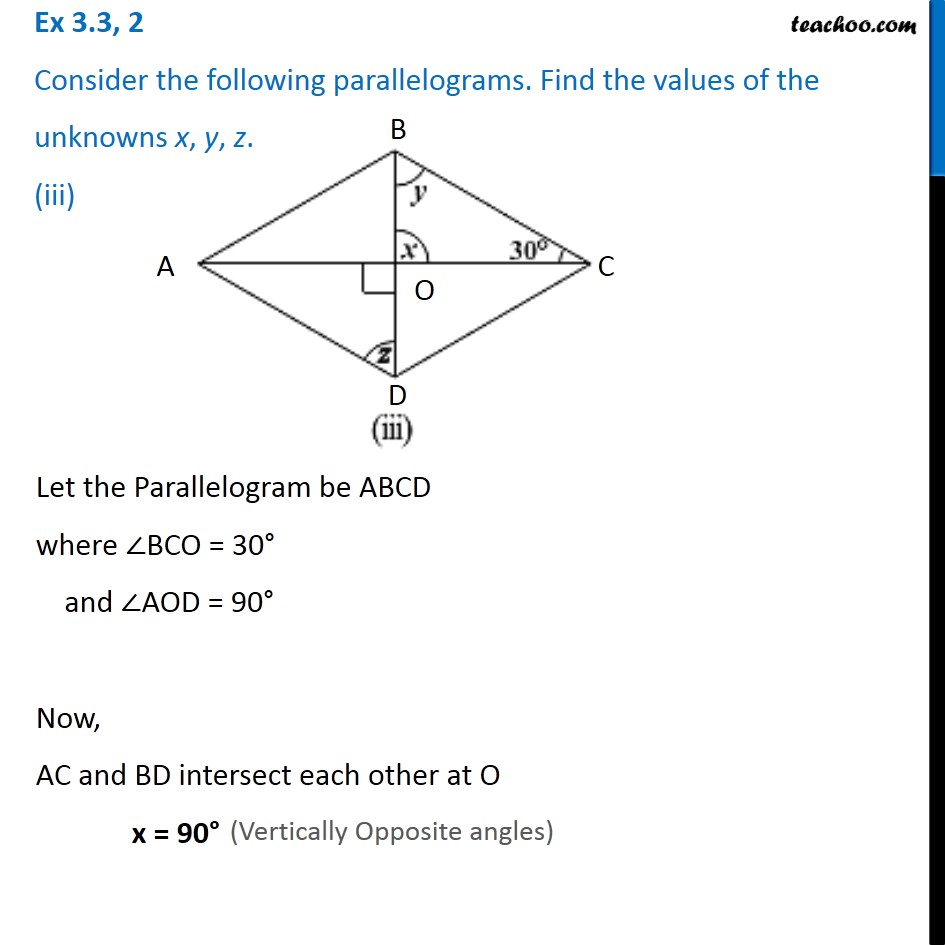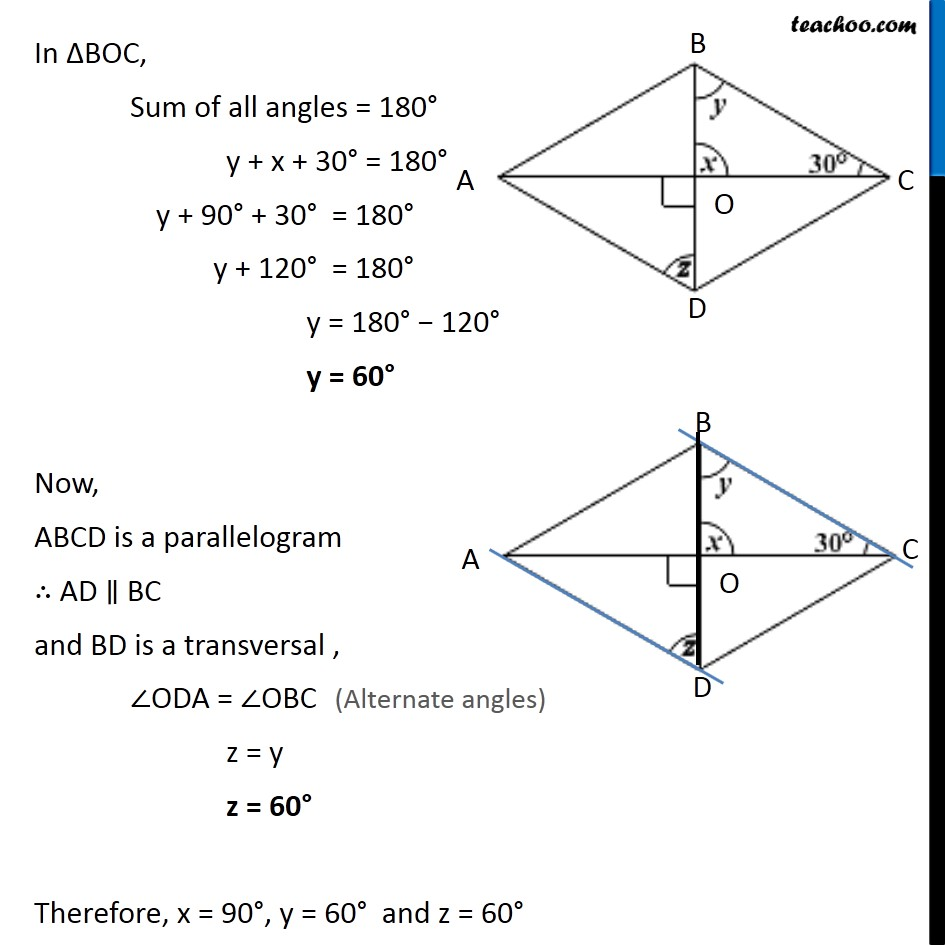Ex 3.3

Chapter 3 Class 8 Understanding Quadrilaterals
Serial order wiseLearn in your speed, with individual attention - Teachoo Maths 1-on-1 Class

### Transcript

Ex 3.3, 2 Consider the following parallelograms. Find the values of the unknowns x, y, z. (iii)Let the Parallelogram be ABCD where ∠BCO = 30° and ∠AOD = 90° Now, AC and BD intersect each other at O x = 90° In ∆BOC, Sum of all angles = 180° y + x + 30° = 180° y + 90° + 30° = 180° y + 120° = 180° y = 180° − 120° y = 60° Now, ABCD is a parallelogram ∴ AD ∥ BC and BD is a transversal , ∠ODA = ∠OBC z = y z = 60° Therefore, x = 90°, y = 60° and z = 60°